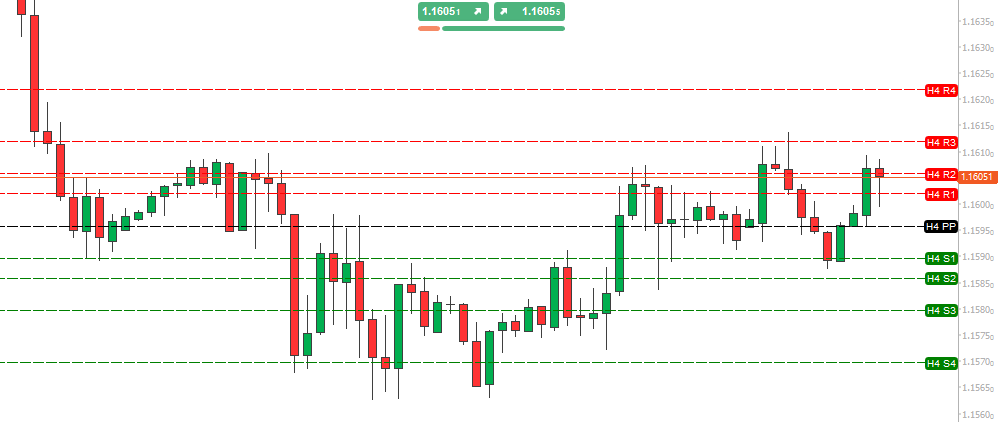This Pivot Point indicator runs on the cTrader trading platform and was originally developed by floor traders in the Forex & Commodities markets to determine potential turning points. many day traders use pivot points to help identify likely levels of support and resistance that show possible turning points from bearish to bullish and vice versa.
Current version: 1.0.0
Requires: Windows 10
Published: 04 October 2021
Page last updated: 04 October 2021

Pivot Points are significant support and resistance levels that can be used to determine potential trades. This version of the indicator has 4-different types of calculations. It is well known that support and resistance levels are key trading tools in any market. There are many formulas for calculating the pivot point (P) of a Forex symbol and the most common Classical is the arithmetic average of the high (H), low (L), and closing (C) prices of the previous trading period:

P = (H + L + C) / 3.

The main pivot point (PP) is the central pivots based on which all other pivot levels are calculated.## Usage Tips

• Pivot points are levels of support, & resistance and can show market turning points.
• Pivot points can show many levels of support and resistance.
• Classical pivot points are calculated using the previous day's high, low & closing prices.
• Pivot points are used as exit points of trades but are rarely used as entry signals.

### INDICATOR FEATURES

• Pivot point periods can be 1-hour, 4-hours, 8-hours, 12-hours, Daily, Weekly & Monthly.
• Four different types of Pivot Point calculations as shown below.
• Up to 4-different levels of support & resistance lines can be shown R1, R2, R3 & R4.
• Option to change the colours of the lines.
• Option to show full, compact or hide the price labels.
• Option to change the pivot lines thickness and type.

## Types of Pivot Point Calculations

This indicator uses 4 different types of calculations for the Pivot Points, you can research on the internet for more information about each one and how you would use it in your trading strategies, but below is a brief explanation of each one to help you get started.

### Classical

This is the most common and most used formula.

P = (H + L + C) / 3.

### Fibonacci

The Fibonacci pivot point levels are defined by first calculating like you would the standard method, but then factoring in the Fibonacci sequence by multiplying the previous day’s range with its corresponding Fibonacci levels. You then subtract the sums you get to the pivot point and you have your Fibonacci pivot point levels.

### Camarilla

This is a modified version of the classic Pivot Point that uses Fibonacci numbers in its calculation of pivot levels and was created in 1989 by Nick Scott who was a successful bond trader. The Camarilla formulas are similar to the Woodie formula but have 8-levels, 4-support and 4-resistance.

### Woody

This uses a very different formula than the classical standard calculation as it uses the difference between the previous day’s high and low.

## How To Install

First, make sure you have the cTrader trading platform installed and then simply unzip the file and double-click on it to automatically install it onto the platform.

## Instant Chat Support

If you want a speedy reply to your questions just post your questions on our Telegram chat group.